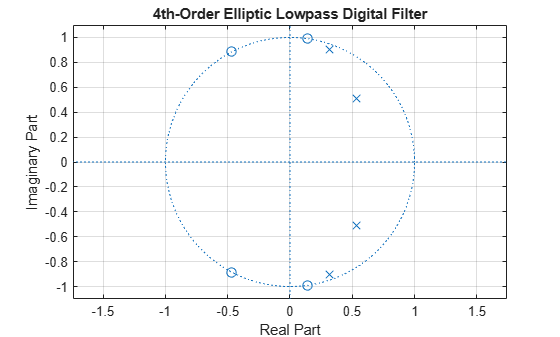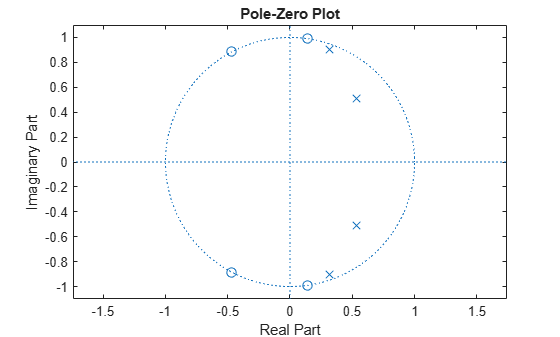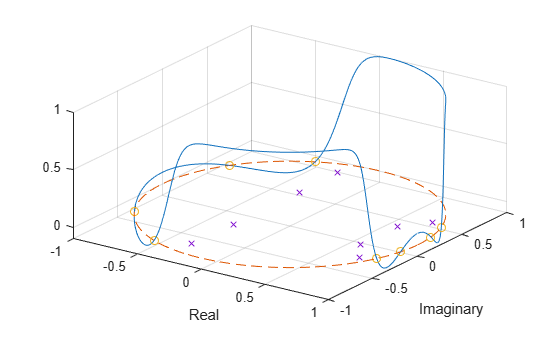zplane

Zero-pole plot for discrete-time systems

Description

example

zplane(z,p) plots the zeros specified in column vector z and the poles specified in column vector p in the current figure window. The symbol 'o' represents a zero and the symbol 'x' represents a pole. The plot includes the unit circle for reference.

If z and p are matrices, then zplane plots the poles and zeros in the columns of z and p in different colors.

example

zplane(b,a), where b and a are row vectors, first uses roots to find the zeros and poles of the transfer function represented by the numerator coefficients b and the denominator coefficients a.

[hz,hp,ht] = zplane(___) returns vectors of handles to the zero lines, hz, and the pole lines, hp. ht is a vector of handles to the axes/unit circle line and to text objects, which are present when there are multiple zeros or poles.

zplane(d) finds the zeros and poles of the transfer function represented by the digital filter, d. Use designfilt to generate d based on frequency-response specifications. The pole-zero plot is displayed in FVTool.

[vz,vp,vk] = zplane(d) returns the zeros (vector vz), poles (vector vp), and gain (scalar vk) corresponding to the digital filter d.

Examples

collapse all

For data sampled at 1000 Hz, plot the poles and zeros of a 4th-order elliptic lowpass digital filter with cutoff frequency 200 Hz, 3 dB of ripple in the passband, and 30 dB of attenuation in the stopband.

[z,p,k] = ellip(4,3,30,200/500);
zplane(z,p)
grid
title('4th-Order Elliptic Lowpass Digital Filter')Create the same filter using designfilt. Use zplane to plot the poles and zeros. Note that this syntax of zplane calls fvtool.

d = designfilt('lowpassiir','FilterOrder',4,'PassbandFrequency',200, ...
'PassbandRipple',3,'StopbandAttenuation',30, ...
'DesignMethod','ellip','SampleRate',1000);
zplane(d)Design an 8th-order Chebyshev Type II bandpass filter with a stopband attenuation of 20 dB. Specify the stopband edge frequencies as $\pi /8$ rad/sample and $5\pi /8$ rad/sample.

[b,a] = cheby2(8/2,20,[1 5]/8);

Use zplane to plot the poles and zeros of the transfer function.

zplane(b,a)Visualize the zero-phase response of the filter. Overlay the unit circle and the pole and zero locations.

[hw,fw] = zerophase(b,a,1024,"whole");

z = roots(b);
p = roots(a);

plot3(cos(fw),sin(fw),hw)
hold on
plot3(cos(fw),sin(fw),zeros(size(fw)),'--')
plot3(real(z),imag(z),zeros(size(z)),'o')
plot3(real(p),imag(p),zeros(size(p)),'x')
hold off
xlabel("Real")
ylabel("Imaginary")
view(35,40)
gridInput Arguments

collapse all

Zeros and poles, specified as column vectors or matrices. If z and p are matrices, then zplane plots the poles and zeros in the columns of z and p in different colors.

Data Types: single | double
Complex Number Support: Yes

Transfer function coefficients, specified as row vectors. The transfer function is defined in terms of z–1:

$H\left(z\right)=\frac{B\left(z\right)}{A\left(z\right)}=\frac{b\left(1\right)+b\left(2\right){z}^{-1}+\cdots +b\left(n+1\right){z}^{-n}}{a\left(1\right)+a\left(2\right){z}^{-1}+\cdots +a\left(m+1\right){z}^{-m}}$

Example: b = [1 3 3 1]/6 and a = [3 0 1 0]/3 specify a third-order Butterworth filter with normalized 3-dB frequency 0.5π rad/sample.

Data Types: single | double
Complex Number Support: Yes

Digital filter, specified as a digitalFilter object. Use designfilt to generate a digital filter based on frequency-response specifications.

Example: d = designfilt('lowpassiir','FilterOrder',3,'HalfPowerFrequency',0.5) specifies a third-order Butterworth filter with normalized 3-dB frequency 0.5π rad/sample.

Output Arguments

collapse all

Vectors of handles to the zero lines, hz, and the pole lines, hp, of the pole-zero plot. ht is a vector of handles to the axes/unit circle line and to text objects, which are present when there are multiple zeros or poles. If there are no zeros or no poles, hz or hp is the empty matrix, [].

Zeros, poles, and gain of a digital filter, d, returned as column vectors and a scalar.

Tips

• You can override the automatic scaling of zplane using

axis([xmin xmax ymin ymax])

after calling zplane. This scaling is useful when one or more zeros or poles have such a large magnitude that the others are grouped tightly around the origin and are hard to distinguish.